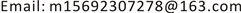1. 引言

2. 树在递归分析中的应用

(1)void Recursive (int m, int n)

{

(2) if (m==0)

(3) return ;

(4) if (n==0)

{

(5) a= Recursive (m-1,1);

(6) return a;

}

(7)else

{

(8) b= Recursive (m-1,n);

(9) printf(“%d %d”,m,n);

(10) c= Recursive (m,n-1);

}

}

2.1. 分析程序结构

2.2. 建立树型图

2.3. 建立状态调用表

3. 实例分析3.1. 汉诺塔问题

void hanoi (int n,char a,char b,char c)

{

if(n==1)

{

printf(“%c-->%c”, a,c);//1

}

else

{

hanoi(n-1,a,c,b );

printf(“%c-->%c”, a,c);//2

hanoi(n-1,b,a,c);

}

}

The Tower of Hanoi call status table on execution procedur

1H(3,’A’,’B’,’C’)
2H(2,’A’,’C’,’B’)
3H(1,’A’,’B’,’C’)‘A’→’C’
H2H(2,’A’,’C’,’B’)回溯到2节点，搜索4节点
‘A’→’B’执行语句2，父节点为2
4H(1,’C’,’A’,’B’)‘C’→’B’
H2H(2,’A’,’C’,’B’)回溯节点2，2节点搜索完毕
H1H(3,’A’,’B’,’C’)回溯到1节点，搜索右分支
‘A’→’C’在5节点前执行
5H(2,’B’,’A’,’C’)
6H(1,’B’,’C’,’A’)‘B’→’A’
H5H(2,’B’,’A’,’C’)回溯节点5
‘B’→’C’执行语句2，父节点为5
7H(1,’A’,’B’,’C’)‘A’→’C’父节点为5
H5H(2,’B’,’A’,’C’)回溯节点5，节点5搜索完毕
H1H(3,’A’,’B’,’C’)回溯到根节点，处理完毕

3.2. 2018 noip普及组试题三(3)

int findans(int n,int m)

{

if(n==0) return m;

if(m==0) return n%3;

return findans(n-1,m)-findans(n,m-1)+findans(n-1,m-1);

}

f1= findans(n-1,m)

f2= findans(n,m-1)

f3= findans(n-1,m-1)

return f1-f2+f3

The call status table of 2018 noip popularization grou

1f(1,2)
2f(0,2)f1 = 2f1 = f(0,2) = 2
H1f(1,2)回溯，搜索第二分支
3f(1,1)
4f(0,1)f1 = 1f1 = f(0,1) = 1
H3f(1,1)回溯，搜索第二分支
5f(1,0)f2 = 1f2 = f(1,0) = 1
H3f(1,1)回溯，搜索第三分支
6f(0,0)f3 = 0f3 = f(0,0) = 0
f2 = f1 − f2 + f3 = 0
H3f(1,1)f2 = 0回溯节点3，搜索完毕
H1f(1,2)回溯，搜索第三分支
7f(0,1)f3 = 1f3 = f(0,1) = 1
f1 − f2 + f3 = 2 – 0 + 1 = 3
H1f(1,2)3回溯到根节点，结束

3.3. 阿克曼函数

n+1m=0,n>0

A(m,n)=A(m-1,1) n=0,m>0

A(m-1,A(m,n-1)) n>0,m>0

int Ackerman(int m, int n)

{

if (m==0)

return n + 1;

if (n==0)

return Ackerman(m - 1, 1);

else

return Ackerman(m - 1,Ackerman(m, n - 1));

}

a= Ackerman(m, n - 1);

b= Ackerman(m - 1,a);

return b;

t1调用树结构如图3所示，执行过程调用状态如表3所示，跳转后的分支树t2的调用树结构如图4所示，执行过程调用状态如表4所示。

The call status table of T

1A(1,2)
2A(1,1)
3A(1,0)执行t2树2接收t2树返回值
H2A(1,1)a = 2回溯节点2
4A(0,2)n + 1 = 3b = 3
H2A(1,1)b = 3回溯节点2
H1A(1,2)3回溯节点1，搜索右分支
5A(0,3)n + 1 = 4b = 4
H1A(1,1)4回溯根节点1，搜索结束

The call status table of T

1A(1,0)
2A(0,1)n + 12
H1A(1,0)2回溯节点1，t2树执行完毕

4. 结论